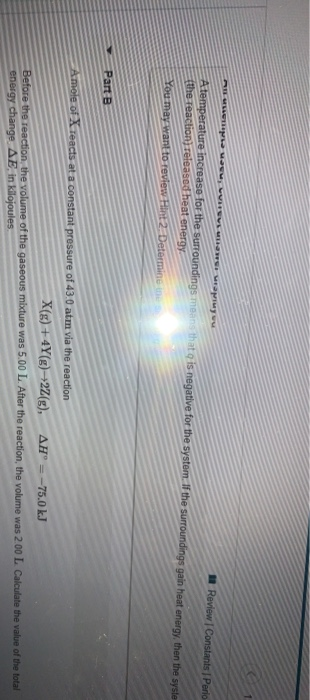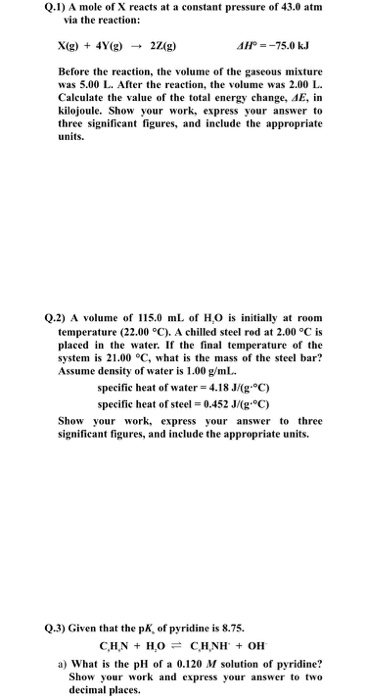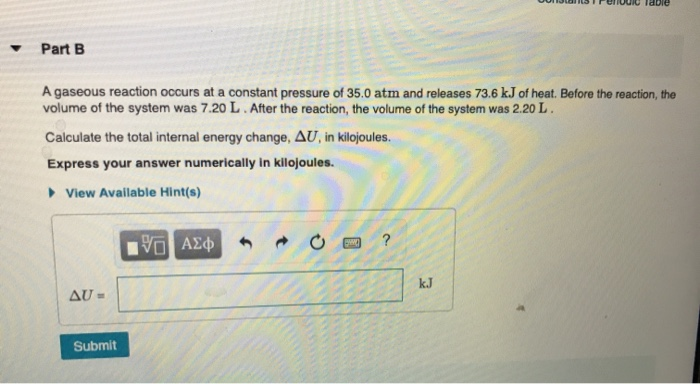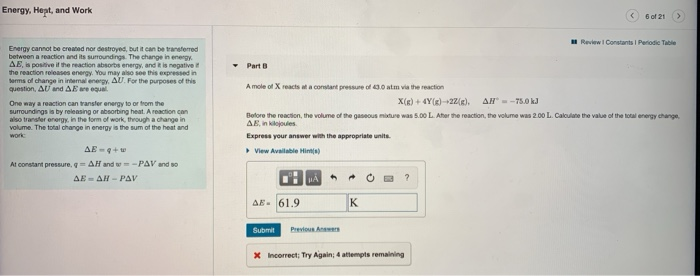Question

# A mole of X reacts at a constant pressure of 43.0 atm via the reaction X(g)+4Y(g)→2Z(g), ΔH∘=−75.0 kJ Before the reacti...

A mole of X reacts at a constant pressure of 43.0 atm via the reaction X(g)+4Y(g)→2Z(g), ΔH∘=−75.0 kJ Before the reaction, the volume of the gaseous mixture was 5.00 L. After the reaction, the volume was 2.00 L. Calculate the value of the total energy change, ΔE, in kilojoules.

Use the first law of thermodynamics:

E = q + w, where q is heat and w is work

For a gas reaction where the only work involved is a change in volume,, we can rewrite this as:

E = q - PV

For a chemical reaction where we care about changes, we can write:

ΔE = ΔH - Δ(PV),

You have a pressure of 43.0 atm and a change from 5 to 2 L. So

Δ(PV) = 43 (2-5) L = -129 L-atm

And converting L atm to J:

129 x 101.325 = -13071 J

Finally:

ΔE = -75.0 - (-13071) = -75.0 kJ + 13.071 kJ = -61.9 kJ

#### Earn Coins

Coins can be redeemed for fabulous gifts.

Similar Homework Help Questions
• ### A mole of X reacts at a constant pressure of 43.0 atm via the reaction X(g)+4Y(g)→2Z(g),    &nbs...

A mole of X reacts at a constant pressure of 43.0 atm via the reaction X(g)+4Y(g)→2Z(g),      ΔH∘=−75.0 kJ Before the reaction, the volume of the gaseous mixture was 5.00 L. After the reaction, the volume was 2.00 L. Calculate the value of the total energy change, ΔE, in kilojoules. Express your answer numerically in kilojoules.

• ### Assuming constant pressure, rank these reactions from most energy released by the system to most energy absorbed by the...

Assuming constant pressure, rank these reactions from most energy released by the system to most energy absorbed by the system, based on the following descriptions: Surroundings get colder and the system decreases in volume. Surroundings get hotter and the system expands in volume. Surroundings get hotter and the system decreases in volume. Surroundings get hotter and the system does not change in volume. Also assume that the magnitude of the volume and temperature changes are similar among the reactions. Rank...

• ### Review Constants Perio A temperature increase for the surroundings mo the reactions released heat energy t...Review Constants Perio A temperature increase for the surroundings mo the reactions released heat energy t s negative for the system. I the surroundings gain heat energy, then the syste You may want to review int Determine Part B Amole of Teacts at a constant pressure of 43.0 atm via the reaction X(g) + 4Y()+22(g), AH = -75.0 kJ Before the reaction, the volume of the gaseous mixture was 5.00 L. After the reaction, the volume was 200 L Calculate...

• ### please solve this on paper wiyh the steps thank u Q.1) A mole of X reacts...please solve this on paper wiyh the steps thank u Q.1) A mole of X reacts at a constant pressure of 43.0 atm via the reaction: X(g) + 4Y(g) + 2/(g) AH° = -75.0 kJ Before the reaction, the volume of the gaseous mixture was 5.00 L. After the reaction, the volume was 2.00 L. Calculate the value of the total energy change, AE, in kilojoule. Show your work, express your answer to three significant figures, and include the appropriate...

• ### 1. Calculate the enthalpy change, ΔH, for the process in which 31.6 g of water is...

1. Calculate the enthalpy change, ΔH, for the process in which 31.6 g of water is converted from liquid at 0.4 ∘C to vapor at 25.0 ∘C . For water, ΔHvap = 44.0 kJ/mol at 25.0 ∘C and Cs = 4.18  J/(g⋅∘C) for H2O(l). 2. How many grams of ice at -16.2 ∘C can be completely converted to liquid at 10.2 ∘C if the available heat for this process is 4.27×103 kJ ? For ice, use a specific heat of 2.01...

• ### DIFCIU idue Part B A gaseous reaction occurs at a constant pressure of 35.0 atm and...DIFCIU idue Part B A gaseous reaction occurs at a constant pressure of 35.0 atm and releases 73.6 kJ of heat. Before the reaction, the volume of the system was 7.20 L. After the reaction, the volume of the system was 2.20 L. Calculate the total internal energy change, AU, in kilojoules. Express your answer numerically in kilojoules. View Available Hint(s) YO AE AU- Submit

• ### part a. The air in an inflated balloon (defined as the system) is warmed over a toaster and absorbs 110 J of heat. As it...

part a. The air in an inflated balloon (defined as the system) is warmed over a toaster and absorbs 110 J of heat. As it expands, it does 79 kJ of work. What is the change in internal energy for the system? Express the energy in kilojoules to two significant figures. part b. When fuel is burned in a cylinder equipped with a piston, the volume expands from 0.235 L to 1.350 L against an external pressure of 1.02 atm...

• ### An ideal gaseous reaction occurs at a constant pressure of 35.0 atm and releases 55.8 kJ...

An ideal gaseous reaction occurs at a constant pressure of 35.0 atm and releases 55.8 kJ of heat. Before the reaction, the volume of the system was 8.20 L. After the reaction, the volume of the system was 3.01 L. Calculate the total change in internal energy for the system.

• ### An ideal gaseous reaction (which is a hypothetical gaseous reaction that conforms to the laws governing gas behavior) oc...

An ideal gaseous reaction (which is a hypothetical gaseous reaction that conforms to the laws governing gas behavior) occurs at a constant pressure of 50.0 atm and releases 65.0 kJ of heat. Before the reaction, the volume of the system was 7.60 L . After the reaction, the volume of the system was 2.20 L . Calculate the total internal energy change, ΔE, in kilojoules. Express your answer with the appropriate units.

• ### Energy, Hept, and Work 621 > Review Constants Periodic Table Energy cannot be created nor destroyed, but it can...Energy, Hept, and Work 621 > Review Constants Periodic Table Energy cannot be created nor destroyed, but it can be transferred between a reaction and its surroundings. The change in energy AE is positive if the reaction absorbs energy, and it is negative the reaction release energy. You may also see this expressed in Dorms of change in internal energy. AU. For the purposes of this question, AU and we gua. One way a reaction can transfer energy to or...